Get instant live expert help with Excel or Google Sheets“My Excelchat expert helped me in less than 20 minutes, saving me what would have been 5 hours of work!”

#### Post your problem and you’ll get Expert help in seconds.

Your message must be at least 40 characters
Our professional Expert are available now. Your privacy is guaranteed.

# Display the current date

While working with Excel, we are able to display the current date by using TODAY or NOW function. This step by step tutorial will assist all levels of Excel users in displaying the current date in three different methods.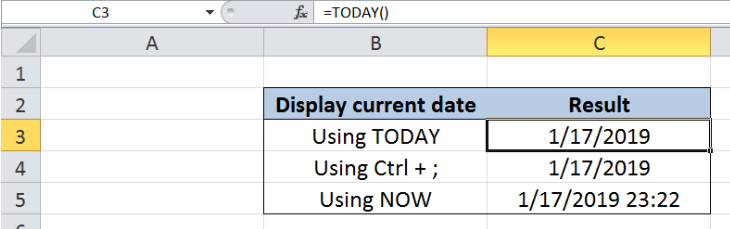Figure 1. Final result: Display the current date

## Syntax of TODAY Function

`=TODAY()`

• TODAY function does not have any arguments
• TODAY returns the current date
• It can be used in combination with other functions and mathematical operations to obtain the desired results

## Syntax of NOW Function

`=NOW()`

• NOW function does not have any arguments
• NOW returns the current date and time

## Setting up our Data

Our data consists of two columns : Display current date (column B) and Result (column C).  Column B contains the three different methods that we will employ to display the current date.  The results will be recorded in column C.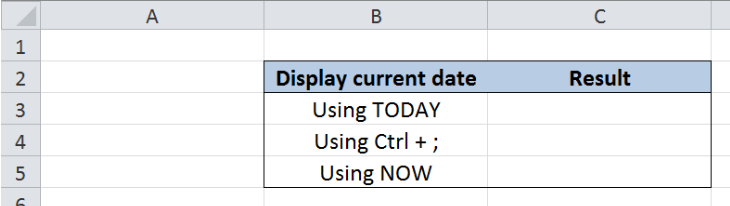Figure 2. Sample template to display current date

## Display current date using TODAY function

Displaying the current date is easily done by using the TODAY function.  Let us follow these steps:

Step 1.  Select cell C3

Step 2.  Enter the formula: `=TODAY()`

Step 3.  Press ENTER

As a result, the value in C3 is 1/17/2019, which is the date today.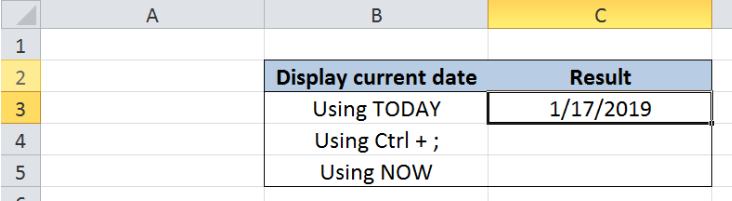Figure 3.  Using TODAY function to display current date

## Display current date using keyboard shortcut

TODAY function returns the current date and recalculates every time the worksheet is opened or updated.  In order to display current date and fix the value at that date, we can make use of the keyboard shortcut Ctrl + ;.

Step 1.  Select cell C4

Step 2.  Press Ctrl + ;

Step 3.  Press ENTER

As a result, the value in C4 is 1/17/2019, which is the same as in our previous example.  The date returned by this method is a constant value and does not update every time the worksheet is opened or updated.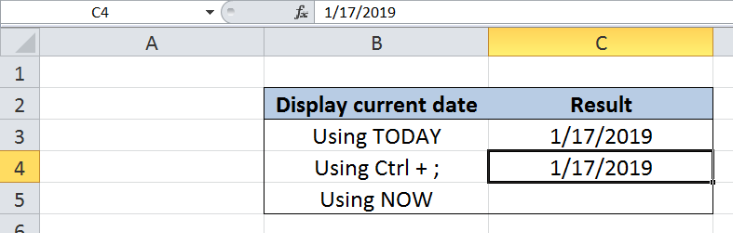Figure 4.  Using Ctrl + ; shortcut to display current date

## Display current date using NOW function

There is a third method to display current date, but this alternative also displays the current time.  Let us follow these steps:

Step 1.  Select cell C5

Step 2.  Enter the formula: `=NOW()`

Step 3.  Press ENTER

As a result, the value in C5 is 1/17/2019 23:24, which is the current date with time.   This function is similar to the TODAY function, wherein it also updates the value every time the worksheet is opened or updated.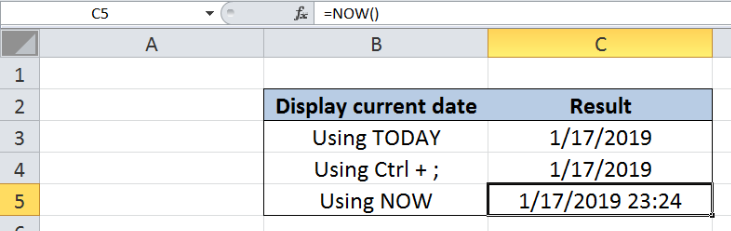Figure 5.  Using NOW function to display current date and time

Most of the time, the problem you will need to solve will be more complex than a simple application of a formula or function. If you want to save hours of research and frustration, try our live Excelchat service! Our Excel Experts are available 24/7 to answer any Excel question you may have. We guarantee a connection within 30 seconds and a customized solution within 20 minutes.

### Did this post not answer your question? Get a solution from connecting with the expert.Another blog reader asked this question today on Excelchat:
Solution examplesI would like my result cell to display 1 of 5 values: If my referenced cell value is less than 2 then the result cell should display 2. (values 0 thru 1.99 = 2) but if my referenced cell value is greater than 2 but less than 2.99 then the new cell should display 3 (values 2 thru 2.99 = 3) but if my referenced cell value is greater than 3 but less than 4.99 then the new cell should display 5 (values 3 thru 4.99 = 5) but if my referenced cell value is greater than 5 but less than 7.5 then the new cell should display 7.5 (values 5 thru 7.5 = 7.5)
Solved by S. Q. in 15 minsi want to insert a multiplication formula in a cell if some percentage value is entered in another cell of same row. for eg. if i put a formula in G7 cell (=G2*e7) but it should be enabled or activated if i put some data entry in f7 cell Excel
Solved by Z. D. in 20 minsDo I have to pay for a solution ? I just wasted time with another help desk that asked for \$46 for an answer. If that is the case, thanks anyway.
Solved by X. U. in 18 minsI would need a formula what copies from my DataInput sheet into another one daily the first two column A and B.
Solved by K. S. in 33 minsI would need a formula what copies from my DataInput sheet into another one daily the first two column A and B.
Solved by B. C. in 60 mins## Subscribe to Excelchat.coAnother blog reader asked this question today on Excelchat: